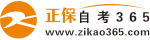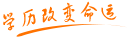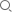#24小时客服：010-82335555# 2004年4月全国高等教育自学考试高等数学基础试题

2005年06月11日    来源：   字体：   打印

课程代码：00417

一、单项选择题（本大题共 30小题，每小题1分，共30分）

在每小题列出的四个备选项中只有一个是符合题目要求的，请将其代码填写在题后的括号内。

1.在空间直角坐标系中，点（-2，1，4）关于x轴的对称点的坐标是（　）

A.（-2，1，-4）； B.（-2，-1，-4）；

C.（2，-1，4）； D.（2，1，-4）；

2.设| |=3，| |=4，且 互相垂直，则| |=（　）

A.0 B.12

C.-12 D.

3.设 是非零向量 的单位向量，则下列各式中成立的是（　）

A.？=| | B.？=

C.？=0 D.？=

4.下列平面中平行于yz面的是（　）

A.y+z=0 B.x+y=0

C.x-5=0 D.y-5=0

5.若平面x+2y-z+3=0与平面kx+4y-2z=0互相平行，则k的值为（　）

A.2 B.-2

C.1 D.-1

6.两直线 和 的夹角为（　）

A. B.？

C.？D.？

7.方程x2+y2+z2-2x+4y-8z-4=0在空间直角坐标系中表示（　）

A.圆 B.球面

C.双曲柱面 D.直圆柱面

8.函数f（x）= 的定义域是（　）

A.（1，+ ） B.[1，+ ）

C.（1，2） D.（2，+ ）

9.下列函数中，在（- ，+ ）内严格递增且函数值大于零的是（　）

A.y=2x B.y=（ ）x

C.y=x2 D.y=x

10.已知an= 则数列{an}（　）

A.无极限 B.以 为极限

C.以2为极限 D.以0为极限

11.在下列函数中，当x 0时，极限值为2的是（　）

A.f（x）= B.f（x）=2sinx

C.f（x）= D.f（x）=

12.函数f（x）在x=x0处有定义是极限 存在的（　）

A.充分条件 B.充分必要条件

C.必要条件 D.无关条件

13.当x 时，下列函数中，为无穷大量的是（　）

A.x2 B.lnx

C.ln（1+x） D.2x

14.x=0是函数f（x）= 的

A.连续点 B.无定义的点

C.可去间断点 D.第二类间断点

15.函数f（x）在x=x0处连续的充要条件是（　）

A. =？=f（x0）

B.？和 都存在

C.？=

D.f（x）在x0处有定义且 存在

16.设f（x）=sinx2，则df（x）=（　）

A.cosx2dx B.sinx2dx

C.2xcosx2dx D.2xsinx2dx

17.设函数y== e-x，则y（n）=（　）

A.ex B.e-x

C.（-1）n-1e-x D.（-1）ne-x

18.函数f（x）=x2-x在[1，3]上满足拉格朗日中值定理的条件，则使f（x）的拉格朗日公式成立的中值 为（？）

A.2 B.1

C.3 D.0

19.函数f（x）=x4在[-1，2]上的最大值是（　）

A.1 B.4

C.16 D.0

20.若 （x）=f（x），x I，则F（x）是f（x）在区间I上的（　）

A.不定积分 B.一个原函数

C.导函数 D.反函数

21.设ex是f（x）的一个原函数，则 （　）

A.ex（x+1）+c B.xex-ex+c

C.ex（1-x）+c D.e-x（x-1）+c

22. 下列各式中，正确的是（　）

A. B.

C. D. +c

23. （　）

A.2[f（x）-f（a）] B.f（2x）-f（2a）

C.2[f（2x）-f（2a）] D.

24.设I= ，则I=（　）

A. B.tanl

C.0 D.1

25.4阶排列2341的逆序数是（　）

A.0 B.1

C.2 D.3

26.行列式 =（　）

A.n！ B.（-1）n+1n！

C.（n-1）！ D.n

27.设A为n阶可逆矩阵，下列等式中一定成立的是（　）

A.（2A）-1=2A-1 B.（2A）T=2AT

C.（（AT）T）-1=（（A-1）-1）T D.（（A-1）-1）T=（AT）-1

28.设A为m n矩阵，且r（A）=r，则下列说法一定正确的是（　）

A.A中r阶子式不全为零 B.A是满秩矩阵

C.A中存在阶数大于r的子式不为零 D.r=min{m，n}

29.线性方程组 的系数矩阵是（　）

A. B.

C. D.

30.设齐次线性方程组Ax=0有n个未知数，其系数矩阵的秩r（A）=r<n，则该方程组的基础解系所含解的个数为（　）

A.n+r B.n-r

C.r D.n

二、填空题（本大题共 10小题，每空1 分，共10分）

31.过点（2，1，-3）且垂直于直线 ___________.

32.直线 的一个方向向量为___________.

33.已知 ___________.

34.已知f（x）= 在x=2处连续，则a=___________.

35.已知 ___________.

36. ___________.

37. ___________.

38.设矩阵A= ， B= ，则BA=___________.

39.若ai5a42a33a21a14是五阶行列式|aij|中的一项，则i=___________.

40.设A是n阶方阵，齐次线性方程组Ax=0有非零解的充分必要条件是|A|=___________.

三、计算题（一）（本大题共4小题，每小题4分，共16分）

41.求过x轴和点（2，-1，3）的平面方程。

42.求极限

43.设函数y=arctan ，求 .

44.已知A= ， B=

求3A-2B

四、计算题（二）（本大题共4小题，每小题7分，共28分）

45.求经过点（2，-3，4）且与直线l1： 和l2： 均垂直的直线方程。

46.设函数y= ，

1） 求该函数的单增区间；2）求该函数的上凸区间。

47.计算？

48.用初等变换判断矩阵A= 是否可逆？

如果可逆，求出逆矩阵。

五、解答题（本大题共2小题，每小题8分，共16分）

49.计算

50.欲用长6m的铝合金料加工一日字形窗框，问长和宽分别为多少时，才能使窗户面积最大，最大面积是多少？